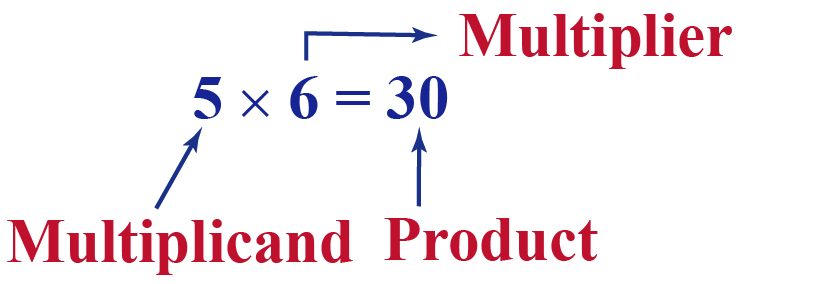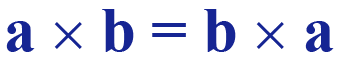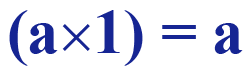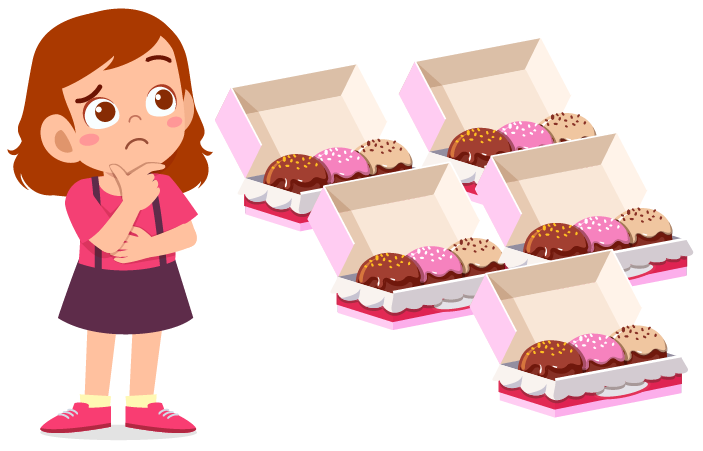# Product in Math

Product in Math

The concept of multiplication is an integral part of math and has a role to play in arithmetic, algebra, and geometry.

In this chapter, you will learn about the product of a number, as well as how to find a product.

Check-out the interactive simulations to know more about the lesson and try your hand at solving a few interesting practice questions at the end of the page.

## Lesson Plan

 1 What is Meant by Product in Math? 2 Tips and Tricks 3 Important Notes on Product in Math 4 Solved Examples on Product in Math 5 Interactive Questions on Product in Math

## What is Meant by Product in Math?

Product in math is defined as the outcome obtained after arithmetic operation of multiplication.

Let us take an example to show the product in math.## How to Find the Product?

The arithmetic operation of multiplication can also be referred to as repetitive addition.

Let's say Joey went out on Halloween and received 3 bags of chocolates, and each bag contains 4 chocolates. How many chocolates does Joey have?The number of chocolates can be calculated in two ways:

• Method 1: He could add the number of chocolates $$4 + 4 + 4 = 12$$
• Method 2: He multiplies the number of chocolates in each bag to tht number of bags, i.e., $$4 \times 3 = 12$$

The answer remains the same in both the cases.

To find the product in math, we always need a multiplicand and a multiplier. Multiplicand represents the number of objects in a group and multiplier represents the number of such equal groups. With reference to the example taken, number of objects in a group was 4 and number of such equal groups was 3Tips and Tricks

• If the order of multiplicand and multiplier are reversed, the product remains the same.
• The product of two positive numbers or two negative numbers always results in a positive number. If any one of them becomes negative, the product becomes negative.

## Explain Product When Different Properties of Multiplication Are Used

There are 4 properties of multiplication:

• Commutative property
• Associative property
• Multiplicative identity property
• Distributive property

### Commutative Property

According to this property of multiplication, the order of the multiplicand and the multiplier does not matter. The product remains the same no matter what is the order.

The property is given asLet's find the product in the example given below.

For example, a = 3 and b = 11

The product of a and b is $$a \times b = 3 \times 11 = 33$$

If the order of a and b is interchanged the product is $$b \times a = 11 \times 3 = 33$$

### Associative Property

When three or more numbers are multiplied together, the product remains the same irrespective of the order of those numbers.

The property is given asLet's see an example.

For example, a = 2, b = 4, and c = 5

The product of a, b, and c is  $$a \times b \times c = 2 \times 4 \times 5 = 40$$

If initially a and b were multiplied and then c was multiplied, the product would be given as $$(a \times b) \times c = (2 \times 4) \times 5 = 8 \times 5 = 40$$

If initially b and c were multiplied and then a was multiplied, the product would be given as $$(b \times c) \times a = (4 \times 5) \times 2 = 20 \times 2 = 40$$

Similarly, If initially a and c were multiplied and then b was multiplied, the product would be given as $$(a \times c) \times b = (2 \times 5) \times 4 = 10 \times 4 = 40$$

### Multiplicative Identity Property

According to this property, any number multiplied by 1 gives the number itself.

The property is given asFor example, when 2 is multiplied by 1, the product is 2, which is the number itself.

### Distributive Property

The sum of any two numbers when multiplied to a third number can be expressed as sum of each one of the addends multiplied by the third number.

The property is given asLet's try finding the product for this case.

For example, $$a = 5, b = 7, and c = 3$$

Applying distributive property, we get $$a \times ( b + c ) = 5 \times ( 7 + 3 ) = 5 \times 10 = 50$$

As per the property, $$(a \times b) + (a \times c) = (5 \times 7) + (5 \times 3) \\= 35 + 15 = 50$$

## Solved Examples

 Example 1

Rihanna has 5 boxes of cupcakes. If 1 box has 3 cupcakes. What is the number of cupcakes she has?Solution

Rihanna knows the multiplicand is 3 and multiplier is 5

Hence, the number of cupcakes she has isequal to the product of 3 and 5, which is 15

 $$\therefore$$ Rihanna has 15 cupcakes.
 Example 2

Justin has to find the product of two numbers such that the multiplicand is the cube of 2 and the multiplier is a square root of 625

Solution

The multiplicand is cube of 2 which is given as $$2^3$$, i.e., 8

The multiplier is a square root of 625 which is given as $$\sqrt{625} = 25$$

The product of the multiplicand and the multiplier is thereby given as $$8 \times 25 = 200$$

 $$\therefore$$ Justin found that the product is 200
 Example 3

What is the product of a number "n" by "(n+1)?" Help Amy find it.

Solution

Amy knows that in this case, number "n" is the multiplicand and "(n+1)" is the multiplier.

The product is given as $$n \times (n+1)$$

Applying distributive property of multiplication, she gets

$$n \times (n+1) \!=\! (n \times n) + (n \times 1) \!=\! n^2 + n$$

 $$\therefore$$ Amy found the product as n2 + n

## Interactive Questions

Here are a few activities for you to practice.

## Let's Summarize

We hope you enjoyed learning about product in math with the practice questions. Now, you will be able to easily solve problems on product in math, find the product, and what is the product of a number.

At Cuemath, our team of math experts is dedicated to making learning fun for our favorite readers, the students!

Through an interactive and engaging learning-teaching-learning approach, the teachers explore all angles of a topic.

Be it worksheets, online classes, doubt sessions, or any other form of relation, it’s the logical thinking and smart learning approach that we, at Cuemath, believe in.

## 1. Which two numbers have a sum of 15 and a product of 36?

The two numbers which have sum 15 and product 36 are 12 and 3

## 2. What is the product of first 50 whole numbers?

The product of first 50 whole numbers is 0

## 3. What is the product of 5 and 7?

The product of 5 and 7 is 35

More Important Topics
More Important Topics
Learn from the best math teachers and top your exams

• Live one on one classroom and doubt clearing
• Practice worksheets in and after class for conceptual clarity
• Personalized curriculum to keep up with school